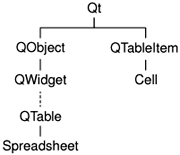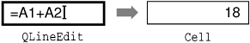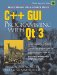# Subclassing QTable

We will now start implementing the Spreadsheet widget, beginning with the header file:

```#ifndef SPREADSHEET_H

#include
#include

class Cell;
```

The header starts with forward declarations for the Cell and SpreadsheetCompare classes.

Figure 4.1. Inheritance tree for Spreadsheet and CellThe attributes of a QTable cell, such as its text and its alignment, are stored in a QTableItem. Unlike QTable, QTableItem isn't a widget class; it is a pure data class. The Cell class is a QTableItem subclass. In addition to the standard QTableItem attributes, Cell stores a cell's formula.

We will explain the Cell class when we present its implementation in the last section of this chapter.

```class Spreadsheet : public QTable
{
Q_OBJECT
public:
Spreadsheet(QWidget *parent = 0, const char *name = 0);

void clear();
QString currentLocation() const;
QString currentFormula() const;
bool autoRecalculate() const { return autoRecalc; }
bool writeFile(const QString &fileName);
QTableSelection selection();
```

The Spreadsheet class inherits from QTable. Subclassing QTable is very similar to subclassing QDialog or QMainWindow.

In Chapter 3, we relied on many public functions in Spreadsheet when we implemented MainWindow. For example, we called clear() from MainWindow::newFile() to reset the spreadsheet. We also used some functions inherited from QTable, notably setCurrentCell() and setShowGrid().

```public slots:
void cut();
void copy();
void paste();
void del();
void selectRow();
void selectColumn();
void selectAll();
void recalculate();
void setAutoRecalculate(bool on);
void findNext(const QString &str, bool caseSensitive);
void findPrev(const QString &str, bool caseSensitive);

signals:
void modified();
```

Spreadsheet provides many slots that implement actions from the Edit, Tools, and Options menus.

```protected:
QWidget *createEditor(int row, int col, bool initFromCell) const;
void endEdit(int row, int col, bool accepted, bool wasReplacing);
```

Spreadsheet reimplements two virtual functions from QTable. These functions are called by QTable itself when the user starts editing the value of a cell. We need to reimplement them to support spreadsheet formulas.

```private:
enum { MagicNumber = 0x7F51C882, NumRows = 999, NumCols = 26 };

Cell *cell(int row, int col) const;
void setFormula(int row, int col, const QString &formula);
QString formula(int row, int col) const;
void somethingChanged();

bool autoRecalc;
};
```

In the class's private section, we define three constants, four functions, and one variable.

```class SpreadsheetCompare
{
public:
bool operator()(const QStringList &row1,
const QStringList &row2) const;

enum { NumKeys = 3 };
int keys[NumKeys];
bool ascending[NumKeys];
};

#endif
```

The header file ends with the SpreadsheetCompare class declaration. We will explain this when we review Spreadsheet::sort().

We will now look at the implementation, explaining each function in turn:

```#include
#include
#include
#include
#include
#include
#include
#include

#include
#include
using namespace std;

#include "cell.h"
```

We include the header files for the Qt classes the application will use. We also include the standard C++ and header files. The using namespace directive imports all the symbols from the std namespace into the global namespace, allowing us to write stable_sort() and vector instead of std::stable_sort() and std::vector.

```Spreadsheet::Spreadsheet(QWidget *parent, const char *name)
: QTable(parent, name)
{
autoRecalc = true;
setSelectionMode(Single);
clear();
}
```

In the constructor, we set the QTable selection mode to Single. This ensures that only one rectangular area in the spreadsheet can be selected at a time.

```void Spreadsheet::clear()
{
setNumRows(0);
setNumCols(0);
setNumRows(NumRows);
setNumCols(NumCols);
for (int i = 0; i < NumCols; i++)
setCurrentCell(0, 0);
}
```

The clear() function is called from the Spreadsheet constructor to initialize the spreadsheet. It is also called from MainWindow::newFile().

We resize the spreadsheet down to 0 x 0, effectively clearing the whole spreadsheet, and resize it again to NumCols x NumRows (26x999). We change the column labels to "A", "B", ..., "Z" (the default is "1", "2", ..., "26") and move the cell cursor to cell A1.

Figure 4.2. QTable's constituent widgetsA QTable is composed of many child widgets. It has a horizontal QHeader at the top, a vertical QHeader on the left, a QScrollBar on the right, and a QScrollBar at the bottom. The area in the middle is occupied by a special widget called the viewport, on which QTable draws the cells. The different child widgets are accessible through functions in QTable and its base class, QScrollView. For example, in clear(), we access the table's top QHeader through QTable:: horizontalHeader().

## Storing Data as Items

In the Spreadsheet application, every non-empty cell is stored in memory as an individual QTableItem object. This pattern of storing data as items is not specific to QTable; Qt's QIconView, QListBox, and QListView classes also operate on items (QIconViewItems, QListBoxItems and QListViewItems).

Qt's item classes can be used out of the box as data holders. For example, a QTableItem already stores a few attributes, including a string, a pixmap, and a pointer back to the QTable. By subclassing the item class, we can store additional data and reimplement virtual functions to use that data.

Many toolkits provide a void pointer in their item classes to store custom data. Qt doesn't burden every item with a pointer that may not be used; instead, it gives programmers the freedom to subclass the item classes and to store the data there, possibly as a pointer to another data structure. If a void pointer is required, it can be trivially achieved by subclassing an item class and adding a void pointer member variable.

With QTable, it is possible to bypass the item mechanism by reimplementing low-level functions such as paintCell() and clearCell(). If the data to display in a QTable is already available in memory in another data structure, this approach can be used to avoid data duplication. For details, see the Qt Quarterly article "A Model/View Table for Large Datasets", available online at http://doc.trolltech.com/qq/qq07-big-tables.html.

Qt 4 is expected to be more flexible than Qt 3 for storing data. In addition to supporting items, Qt 4 will probably offer a single unified item type usable by all item views, and the item views will not take ownership of the items they display, making it possible to display the same items in multiple views simultaneously.

QScrollView is the natural base class for widgets that can present lots of data. It provides a scrollable viewport and two scroll bars, which can be turned on and off. It is covered in Chapter 6.

```Cell *Spreadsheet::cell(int row, int col) const
{
return (Cell *)item(row, col);
}
```

The cell() private function returns the Cell object for a given row and column. It is almost the same as QTable::item(), except that it returns a Cell pointer instead of a QTableItem pointer.

```QString Spreadsheet::formula(int row, int col) const
{
Cell *c = cell(row, col);
if (c)
return c->formula();
else
return "";
}
```

The formula() private function returns the formula for a given cell. If cell() returns a null pointer, the cell is empty, so we return an empty string.

```void Spreadsheet::setFormula(int row, int col,
const QString &formula)
{
Cell *c = cell(row, col);
if (c) {
c->setFormula(formula);
updateCell(row, col);
} else {
setItem(row, col, new Cell (this, formula));
}
}
```

The setFormula() private function sets the formula for a given cell. If the cell already has a Cell object, we reuse it and call updateCell() to tell QTable to repaint the cell if it's shown on screen. Otherwise, we create a new Cell object and call QTable::setItem() to insert it into the table and repaint the cell. We don't need to worry about deleting the Cell object later on; QTable takes ownership of the cell and will delete it automatically at the right time.

```QString Spreadsheet::currentLocation() const
{
return QChar('A' + currentColumn())
+ QString::number(currentRow() + 1);
}
```

The currentLocation() function returns the current cell's location in the usual spreadsheet format of column letter followed by row number. MainWindow::updateCellIndicators() uses it to show the location in the status bar.

```QString Spreadsheet::currentFormula() const
{
return formula(currentRow(), currentColumn());
}
```

The currentFormula() function returns the current cell's formula. It is called from MainWindow::updateCellIndicators().

```QWidget *Spreadsheet::createEditor(int row, int col,
bool initFromCell) const
{
QLineEdit *lineEdit = new QLineEdit(viewport());
lineEdit->setFrame(false);
if (initFromCell)
lineEdit->setText(formula(row, col));
return lineEdit;
}
```

The createEditor() function is reimplemented from QTable. It is called when the user starts editing a celleither by clicking the cell, pressing F2, or simply starting to type. Its role is to create an editor widget to be shown on top of the cell. If the user clicked the cell or pressed F2 to edit the cell, initFromCell is true and the editor must start with the current cell's content. If the user simply started typing, the cell's previous content is ignored.

The default behavior of this function is to create a QLineEdit and initialize it with the cell's text if initFromCell is true. We reimplement the function to show the cell's formula instead of the cell's text.

We create the QLineEdit as a child of the QTable's viewport. QTable takes care of resizing the QLineEdit to match the cell's size and of positioning it over the cell that is to be edited. QTable also takes care of deleting the QLineEdit when it is no longer needed.

Figure 4.3. Editing a cell by superimposing a QLineEditIn many cases, the formula and the text are the same; for example, the formula "Hello" evaluates to the string "Hello", so if the user types "Hello" into a cell and presses Enter, that cell will show the text "Hello". But there are some exceptions:

• If the formula is a number, it is interpreted as such. For example, the formula "1.50" evaluates to the double value 1.5, which is rendered as a right-aligned "1.5" in the spreadsheet.
• If the formula starts with a single quote, the rest of the formula is interpreted as text. For example, the formula "'12345" evaluates to the string "12345".
• If the formula starts with an equals sign ('='), the formula is interpreted as an arithmetic formula. For example, if cell A1 contains "12" and cell A2 contains "6", the formula "=A1+A2" evaluates to 18.

The task of converting a formula into a value is performed by the Cell class. For the moment, the important thing to bear in mind is that the text shown in the cell is the result of evaluating the formula, not the formula itself.

```void Spreadsheet::endEdit(int row, int col, bool accepted,
bool wasReplacing)
{
QLineEdit *lineEdit = (QLineEdit *)cellWidget(row, col);
if (!lineEdit)
return;
QString oldFormula = formula(row, col);
QString newFormula = lineEdit->text();

QTable::endEdit(row, col, false, wasReplacing);

if (accepted && newFormula != oldFormula) {
setFormula(row, col, newFormula);
somethingChanged();
}
}
```

The endEdit() function is reimplemented from QTable. It is called when the user has finished editing a cell, either by clicking elsewhere in the spreadsheet (which confirms the edit), by pressing Enter (which also confirms the edit), or by pressing Esc (which rejects the edit). The function's purpose is to transfer the editor's content back into the Cell object if the edit is confirmed.

The editor is available from QTable::cellWidget(). We can safely cast it to a QLineEdit since the widget we create in createEditor() is always a QLineEdit.

Figure 4.4. Returning a QLineEdit's content to a cellIn the middle of the function, we call QTable's implementation of endEdit(), because QTable needs to know when editing has finished. We pass false as third argument to endEdit() to prevent it from modifying the table item, since we want to create or modify it ourselves. If the new formula is different from the old one, we call setFormula() to modify the Cell object and call somethingChanged().

```void Spreadsheet::somethingChanged()
{
if (autoRecalc)
recalculate();
emit modified();
}
```

The somethingChanged() private function recalculates the whole spreadsheet if Auto-recalculate is enabled and emits the modified() signal.C++ GUI Programming with Qt 3
ISBN: 0131240722
EAN: 2147483647
Year: 2006
Pages: 140